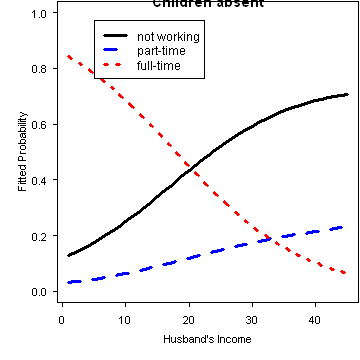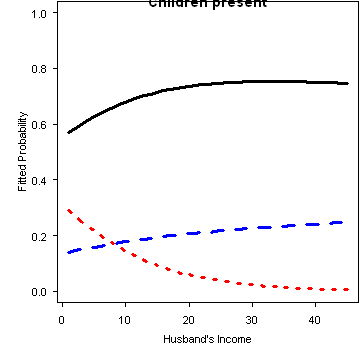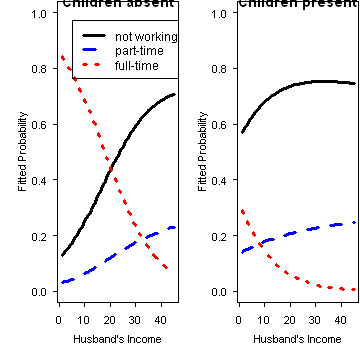# Output from wlf-glogit.R

```# multinomial logistic regression

library(car)
data(Womenlf)
attach(Womenlf)

participation <- ordered(partic, levels = c("not.work", "parttime", "fulltime"))
library(nnet)
mod.multinom <- multinom(participation ~ hincome + children)
```
```## # weights:  12 (6 variable)
## initial  value 288.935032
## iter  10 value 211.454772
## final  value 211.440963
## converged
```
```summary(mod.multinom, Wald = TRUE)
```
```## Call:
## multinom(formula = participation ~ hincome + children)
##
## Coefficients:
##          (Intercept)   hincome childrenpresent
## parttime      -1.432  0.006894         0.02146
## fulltime       1.983 -0.097232        -2.55861
##
## Std. Errors:
##          (Intercept) hincome childrenpresent
## parttime      0.5925 0.02345          0.4690
## fulltime      0.4842 0.02810          0.3622
##
## Value/SE (Wald statistics):
##          (Intercept) hincome childrenpresent
## parttime      -2.418  0.2939         0.04574
## fulltime       4.095 -3.4607        -7.06407
##
## Residual Deviance: 422.9
## AIC: 434.9
```
```Anova(mod.multinom)
```
```## Analysis of Deviance Table (Type II tests)
##
## Response: participation
##          LR Chisq Df Pr(>Chisq)
## hincome      15.2  2    0.00051 ***
## children     63.6  2    1.6e-14 ***
## ---
## Signif. codes:  0 '***' 0.001 '**' 0.01 '*' 0.05 '.' 0.1 ' ' 1
```
```predictors <- expand.grid(hincome = 1:45, children = c("absent", "present"))
p.fit <- predict(mod.multinom, predictors, type = "probs")

Hinc <- 1:max(hincome)
plot(range(hincome), c(0, 1), type = "n", xlab = "Husband's Income", ylab = "Fitted Probability",
main = "Children absent")
lines(Hinc, p.fit[Hinc, "not.work"], lty = 1, lwd = 3, col = "black")
lines(Hinc, p.fit[Hinc, "parttime"], lty = 2, lwd = 3, col = "blue")
lines(Hinc, p.fit[Hinc, "fulltime"], lty = 3, lwd = 3, col = "red")

legend(5, 0.97, lty = 1:3, lwd = 3, col = c("black", "blue", "red"), legend = c("not working",
"part-time", "full-time"))
``````plot(range(hincome), c(0, 1), type = "n", xlab = "Husband's Income", ylab = "Fitted Probability",
main = "Children present")
lines(Hinc, p.fit[46:90, "not.work"], lty = 1, lwd = 3, col = "black")
lines(Hinc, p.fit[46:90, "parttime"], lty = 2, lwd = 3, col = "blue")
lines(Hinc, p.fit[46:90, "fulltime"], lty = 3, lwd = 3, col = "red")
``````
# a more general way to make the plot
op <- par(mfrow = c(1, 2))
Hinc <- 1:max(hincome)
for (kids in c("absent", "present")) {
data <- subset(data.frame(predictors, p.fit), children == kids)
plot(range(hincome), c(0, 1), type = "n", xlab = "Husband's Income", ylab = "Fitted Probability",
main = paste("Children", kids))
lines(Hinc, data[, "not.work"], lwd = 3, col = "black", lty = 1)
lines(Hinc, data[, "parttime"], lwd = 3, col = "blue", lty = 2)
lines(Hinc, data[, "fulltime"], lwd = 3, col = "red", lty = 3)
if (kids == "absent") {
legend(5, 0.97, lty = 1:3, lwd = 3, col = c("black", "blue", "red"), legend = c("not working",
"part-time", "full-time"))
}
}
``````par(op)

detach(Womenlf)
```

Generated with `R version 2.15.1 (2012-06-22)` using the R package knitr (version `0.8.4`) on `Wed Sep 26 09:01:52 2012`.